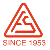﻿ 压缩机常用公制 英制单位转换--上海复盛空压机销售公司|空压机|上海空压机|复盛空压机|空气压缩机|空压机网# 压缩机常用公制 英制单位转换

[复盛空压机] [空压机] [2007-11-16]

Capacity 流量

1 cfm = 0.0283M3/min

1 scfm = 0.0283Nm3/min

Pressure 压力

1 bar= 14.56 pis = 0.1MPa

1 barg= 14.56 pisg = 0.1MPag

Linear Measure 长度

1 inch 英寸=25.4 millimetres 毫米

1 foot 英尺=12 inches 英寸=0.3048 metre 米

1 yard 码=3 feet 英尺=0.9144 metre 米

Square Measure 面积

1 square inch 平方英寸=6.45 sq.centimetres 平方厘米

1 square foot 平方英尺=144 sq.in.平方英寸

=9.29 sq.decimetres 平方分米

Cubic Measure 体积

1 cubic inch 立方英寸=16.4 cu.centimetres 立方厘米

1 cubic foot 立方英尺=1728 cu.in. 立方英寸

=0.0283 cu.metre 立方米

1 cubic yard 立方码=27 cu.ft. 立方英尺

=0.765 cu.metre 立方米

Capacity Measure 容积

Britich 英制

1 quart 夸脱=2 pints 品脱=1.136 litres 升

1 gallon 加伦=4 quarts 夸脱=4.546 litres 升

American liquid 美制液量

1 gallon 加伦=4 quarts 夸脱=3.785 litres 升

1 pound 磅=16 ounces 盎司=7000 grains 谷

=0.4536 kilogram 千克

24小时咨询电话：13681906406  束先生